Numerical study of solutions to sedimentation problems of the Niulan River-Dian Lake Water Supplementation Project
ZHANG Ruoyin, WU BaoshengState Key Laboratory of Hydroscience and Engineering, Tsinghua University, Beijing 100084, China
Abstract: The Deze Reservoir is the water source for the Niulan River-Dian Lake Water Supplementation Project. The very fine incoming sediment and the complicated flow and sediment transport processes in the reservoir have led to high sediment concentrations and turbid water in the intake during floods, which has an adverse impact on the water environment of Dian Lake, Kunming Waterfall Park and Panlong River. This study investigated the effects of two solutions for reducing the intake sediment concentration by three-dimensional modeling. One solution is the construction of check dams in the mainstream and its tributaries while the other is the construction of a new multi-level water intake. The simulations show that the new multi-level water intake designed based on the stratification characteristics of the sediment concentration will increase the length of time until the sediment concentration exceeds the desired limits. The modified intake design keeps the surface layer turbidities to lower than 17.1 NTU during the flood season for between 47.0% and 62.1% of the time surface layer turbidities larger than 100 NTU for less than 7.6% of the time. The check dams will accelerate the deposition upstream of the check dams and reduce the water turbidity at the existing water intake; however, the turbidity is still high with the surface turbidity below the limit for only 13.9%-18.5% of the flood season, which are not much better than the values without the check dams. Thus, the multi-level water intake is the better choice for solving the sediment problems of the Niulan River-Dian Lake Water Supplementation Project because it provides longer periods of low turbidity than the check dams.
Key words: Deze Reservoir     check dam     multi-level water intake     sediment concentration     numerical simulation

SCHISM三维水沙数学模型[15-16]能够满足河流—河口—海洋跨尺度计算的要求，适应剧烈变化的地形条件，在维持较高计算精度的同时采用高性能并行计算的程序架构，大幅度提高计算效率。该模型已成功运用于美国切萨皮克湾、波罗的海和北海连接区、葡萄牙海岸系统等沿海地区和黄河下游河道的计算，具有较好的适用性和准确性。考虑到德泽水库地形复杂及入库泥沙较细的特点，同时为便于更好地描述德泽水库的异重流现象，本文采用SCHISM模型研究不同典型年的汛期水沙过程在德泽水库中的运动规律，对比分析修建拦沙坝前、后沿程及取水口分层含沙量的变化情况，探讨修建拦沙坝以及新建分层取水口方案对降低取水口含沙量的影响效果。研究成果既可为制定牛栏江滇池补水工程汛期泥沙问题的合理治理方案提供参考，又对研究细颗粒泥沙河道中修建拦沙坝的作用及取水口布置等具有重要意义。

1 水库三维水沙数学模型 1.1 模型简介 1.1.1 基本方程

 $\frac{\partial u}{\partial x}+\frac{\partial v}{\partial y}+\frac{\partial w}{\partial z}=0$ (1)
 \begin{aligned} \frac{\mathrm{D} u}{\mathrm{D} t}=& f v-g \frac{\partial \eta}{\partial x}-\frac{1}{\rho_{0}} \frac{\partial p_{A}}{\partial x}-\frac{g}{\rho_{0}} \int_{z}^{\eta} \frac{\partial \rho}{\partial x} d z+\\ & \frac{\partial}{\partial z}\left(K_{\mathrm{mv}} \frac{\partial u}{\partial z}\right)+K_{\mathrm{mh}}\left(\frac{\partial^{2} u}{\partial x^{2}}+\frac{\partial^{2} u}{\partial y^{2}}\right), \end{aligned} (2)
 \begin{aligned} \frac{\mathrm{D} v}{\mathrm{D} t}=&-f u-g \frac{\partial \eta}{\partial y}-\frac{1}{\rho_{0}} \frac{\partial p_{\mathrm{A}}}{\partial y}-\frac{g}{\rho_{0}} \int_{z}^{\eta} \frac{\partial \rho}{\partial y} d z+\\ & \frac{\partial}{\partial z}\left(K_{\mathrm{mv}} \frac{\partial v}{\partial z}\right)+K_{\mathrm{mh}}\left(\frac{\partial^{2} v}{\partial x^{2}}+\frac{\partial^{2} v}{\partial y^{2}}\right). \end{aligned} (3)

 $\frac{\partial \eta}{\partial t}+\frac{\partial}{\partial x} \int_{z_{{\rm b}}}^{\eta} u \mathrm{d} z+\frac{\partial}{\partial y} \int_{z_{{\rm b}}}^{\eta} v \mathrm{d} z=0.$ (4)

 $K_{\mathrm{mv}}\left(\frac{\partial u}{\partial z}, \frac{\partial v}{\partial z}\right)=\left(\tau_{b x}, \tau_{b y}\right), z=z_{\mathrm{b}}.$ (5)

1.1.2 泥沙模型

 $\frac{\partial C}{\partial t}+\frac{\partial(u C)}{\partial x}+\frac{\partial(v C)}{\partial y}+\frac{\partial\left[\left(w-\omega_{s}\right) C\right]}{\partial z}=\\ \frac{\partial}{\partial z}\left(K_{\mathrm{sv}} \frac{\partial C}{\partial z}\right)+K_{\mathrm{sh}}\left(\frac{\partial^{2} C}{\partial x^{2}}+\frac{\partial^{2} C}{\partial y^{2}}\right).$ (6)

 $\left[\omega_{\mathrm{s}} C+K_{\mathrm{sv}} \frac{\partial C}{\partial z}\right]=0.$ (7)

 $\left[\omega_{{\rm s}} C+K_{\mathrm{sv}} \frac{\partial C}{\partial z}\right]=\omega_{\mathrm{s}}\left(C_{\mathrm{b}}-\alpha_{*} S_{*}\right).$ (8)

 $C_{\mathrm{b}}=C_{\mathrm{m}}+\left[1-\exp \left(-\frac{\omega_{\mathrm{s}}}{K_{\mathrm{sv}}}\left(z_{\mathrm{m}}-z_{0}\right)\right)\right] \alpha_{*} S_{*}.$ (9)

 $S_{*}=2.5 \times\left[\frac{\left(0.0022+C_{\mathrm{V}}\right) U^{3}}{\kappa_{\mathrm{m}} \frac{\gamma_{\mathrm{s}}-\gamma_{\mathrm{m}}}{\gamma_{\mathrm{m}}} g h \omega_{\mathrm{m}}} \ln \left(\frac{h}{6 D_{50}}\right)\right]^{0.62}.$ (10)

 $\rho^{\prime} \frac{\partial Z}{\partial t}=\omega_{{\rm s}}\left(C_{{\rm b}}-\alpha_{*} S_{*}\right).$ (11)

1.2 研究对象 1.2.1 计算区域与网格划分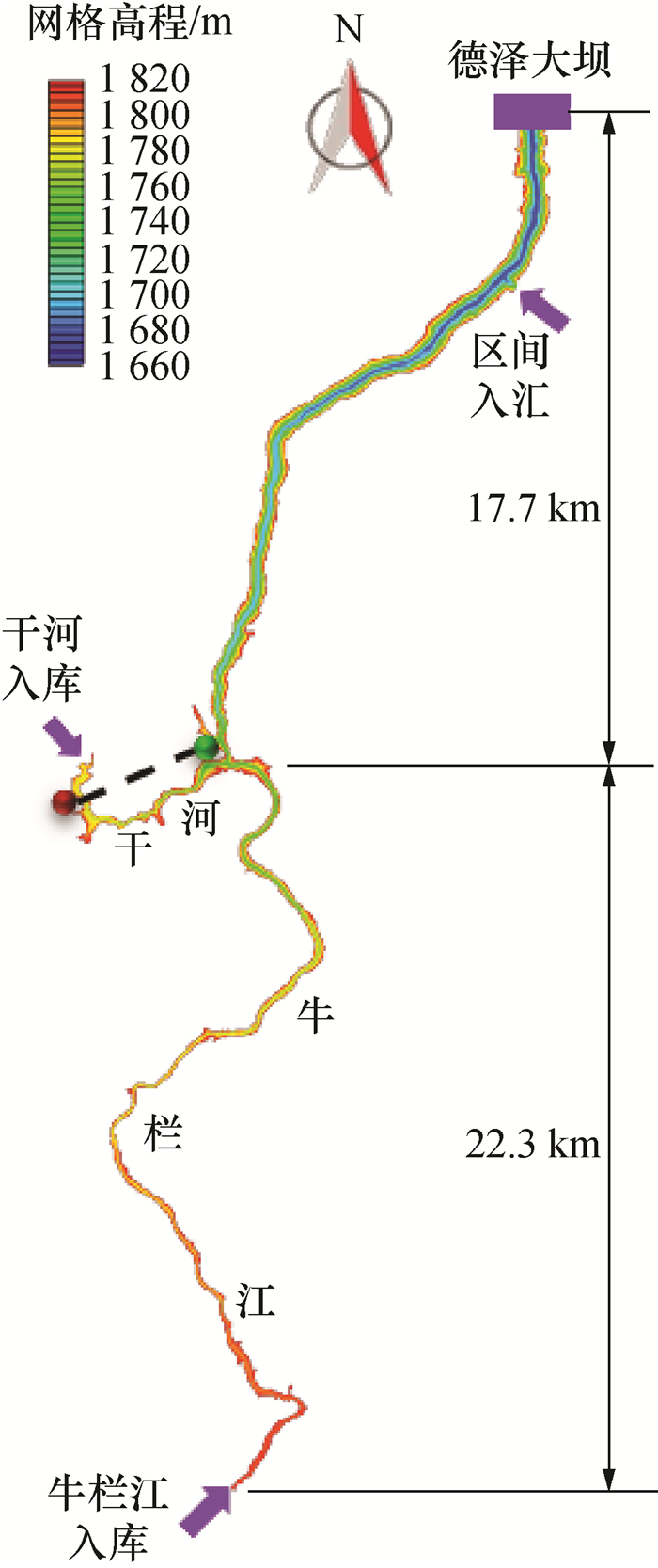图 1 计算水库初始地形条件图 2 干、支流交汇区域与取水口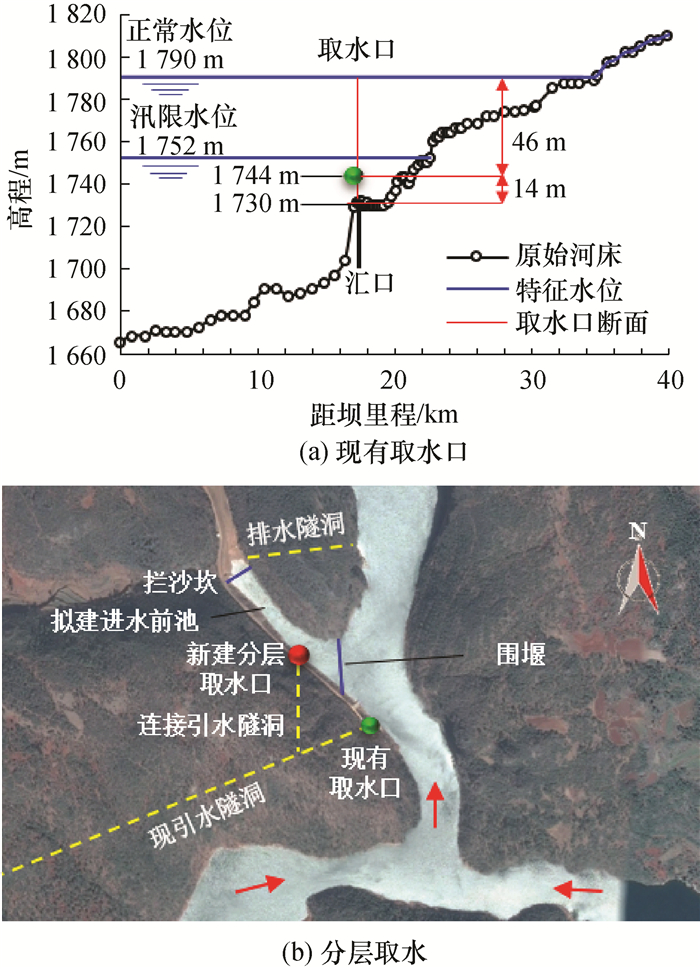图 3 现有取水口与分层取水口

1.2.2 边界条件与相关参数

 代表粒径/mm 入库悬移质占比/% 床沙占比/% 沉速/(mm·s-1) 牛栏江 干河 暗河 牛栏江 干河 0.001 14.51 22.59 14.51 0 0 6.24×10-4 0.006 39.07 41.35 39.07 0 0 2.26×10-2 0.012 23.96 18.01 23.96 0 0 9.07×10-2 0.02 13.21 10.46 13.21 0 0 2.52×10-1 0.06 9.25 7.59 9.25 100 100 2.26

1.3 模型验证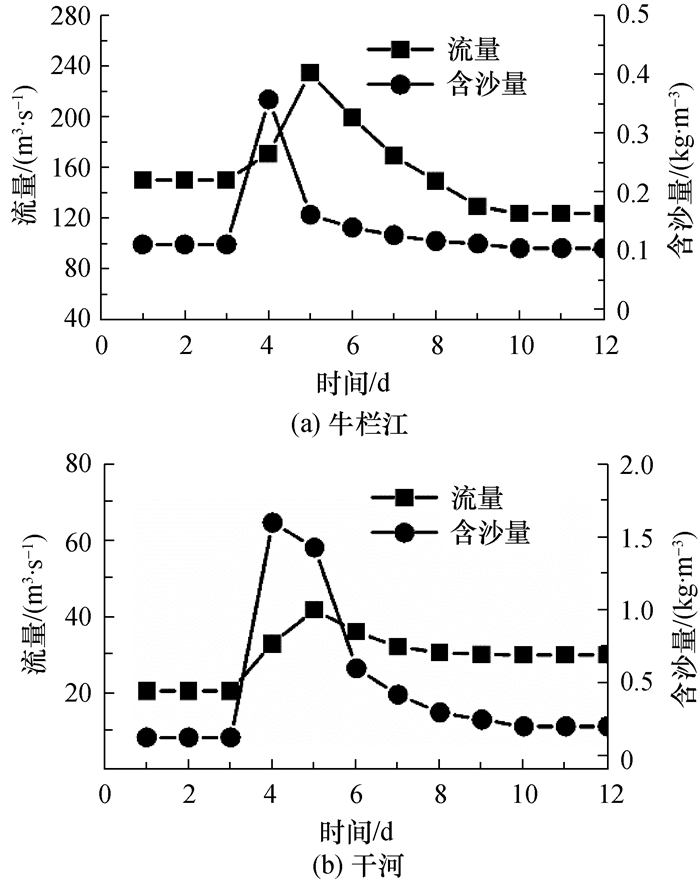图 4 验证计算进口水沙条件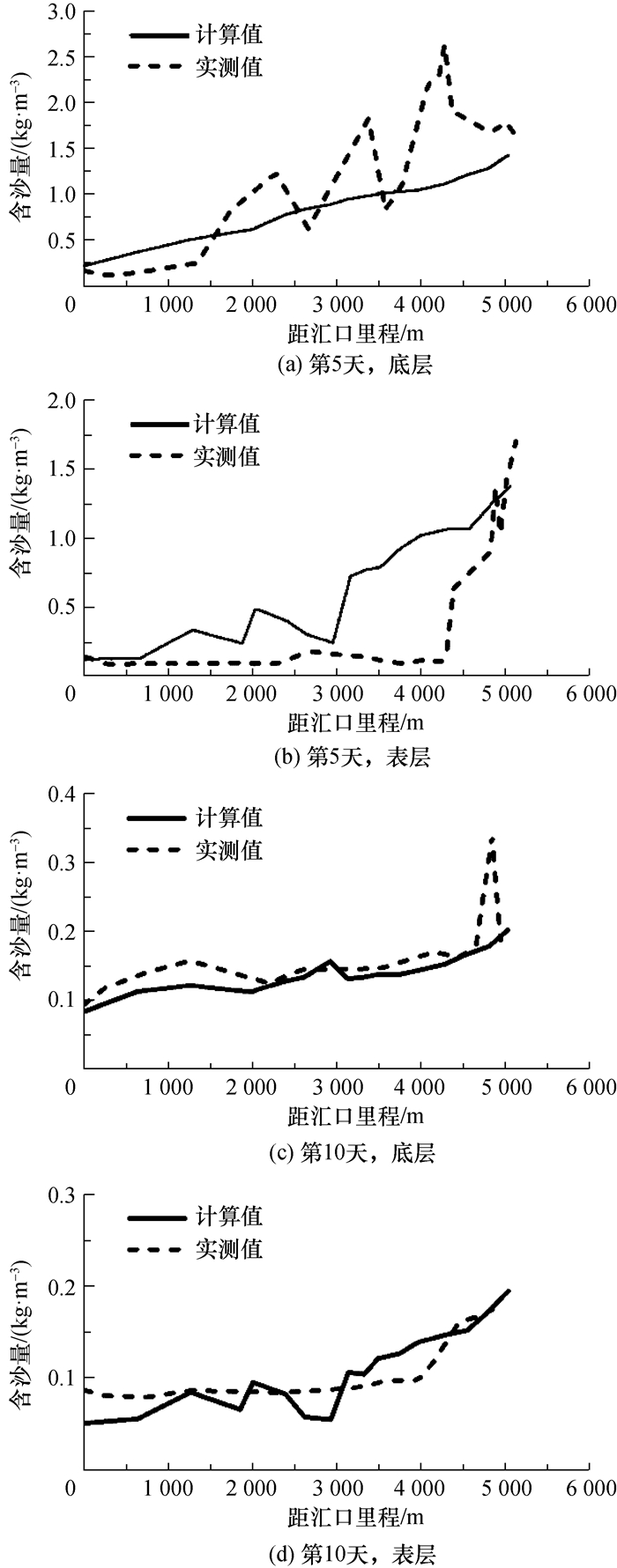图 5 计算与实测干河沿程表层、底层含沙量对比

2 方案模拟结果分析

2.1 干、支流拦沙坝的拦沙效果

 $\phi_{\mathrm{aver}}=\frac{W_{\mathrm{sin}}-W_{\mathrm{sout}}}{W_{\mathrm{sin}}} \times 100 \%.$ (12)

 % 拦沙坝情况 入库—拦沙坝库段 水沙条件 大水大沙 中水中沙 小水小沙 无拦沙坝 干河 35.25 42.73 45.66 牛栏江 34.02 38.90 42.17 有拦沙坝 干河 41.46 45.40 49.93 牛栏江 51.34 53.95 58.02 增加比例 干河 6.21 2.67 4.27 牛栏江 17.32 15.05 15.85

2.2 水库沿程含沙量分布特点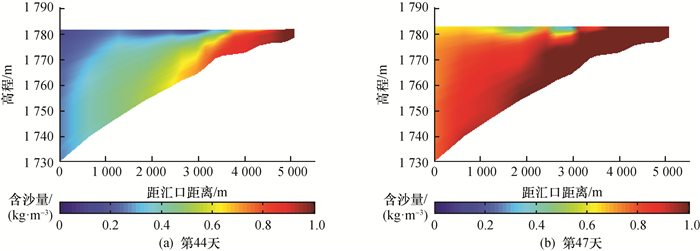图 6 干河无坝时中水中沙年沿程含沙量分布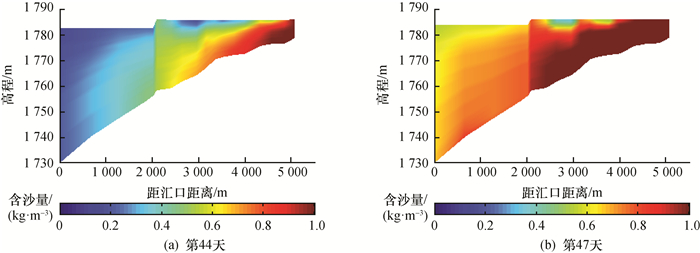图 7 干河有坝时中水中沙年沿程含沙量分布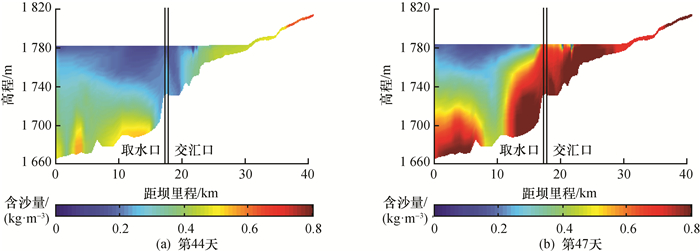图 8 牛栏江无坝时中水中沙年沿程含沙量分布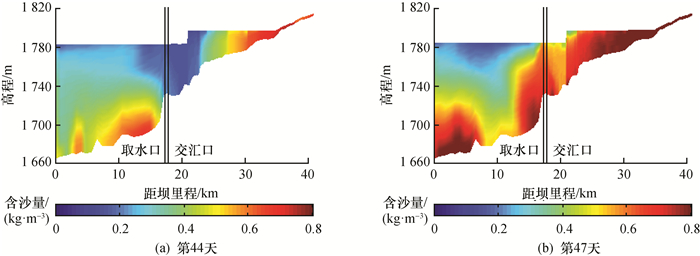图 9 牛栏江有坝时中水中沙年沿程含沙量分布

2.2.1 干河沿程含沙量分布

2.2.2 牛栏江沿程含沙量分布

2.3 取水口分层含沙量变化特点

 项目 拦沙坝情况 水沙条件 大水大沙 中水中沙 小水小沙 断面平均含沙量/(kg·m-3) 无拦沙坝 0.28 0.21 0.24 有拦沙坝 0.19 0.14 0.15 减少比例/% 32.9 31.5 36.7 中层含沙量/(kg·m-3) 无拦沙坝 0.27 0.21 0.24 有拦沙坝 0.15 0.12 0.14 减少比例/% 45.7 42.2 41.4 表层含沙量/(kg·m-3) 无拦沙坝 0.07 0.06 0.07 有拦沙坝 0.12 0.10 0.10 减少比例/% -63.5 -59.2 -34.9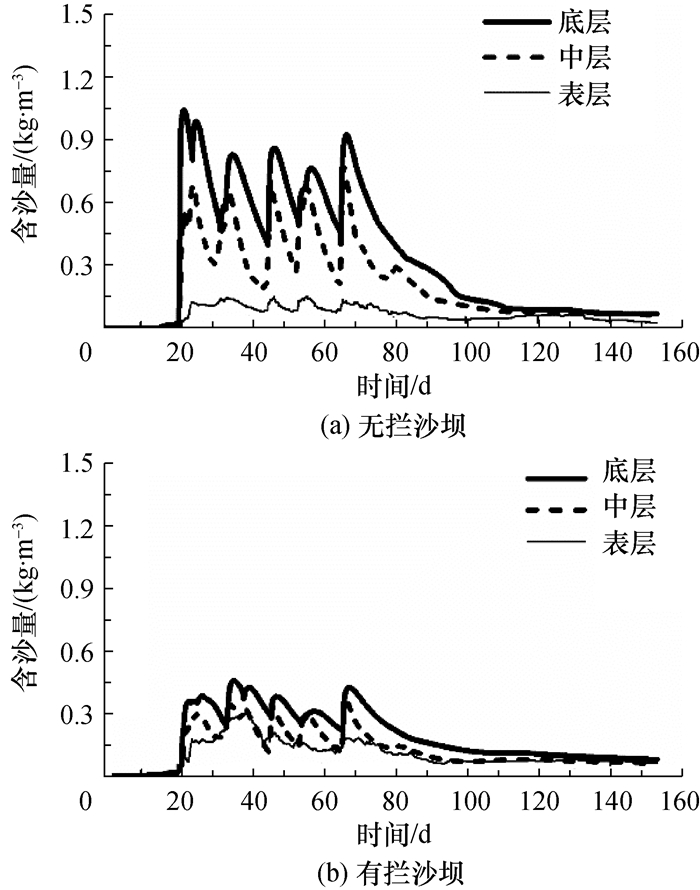图 10 中水中沙年有、无拦沙坝时取水口断面分层含沙量随时间变化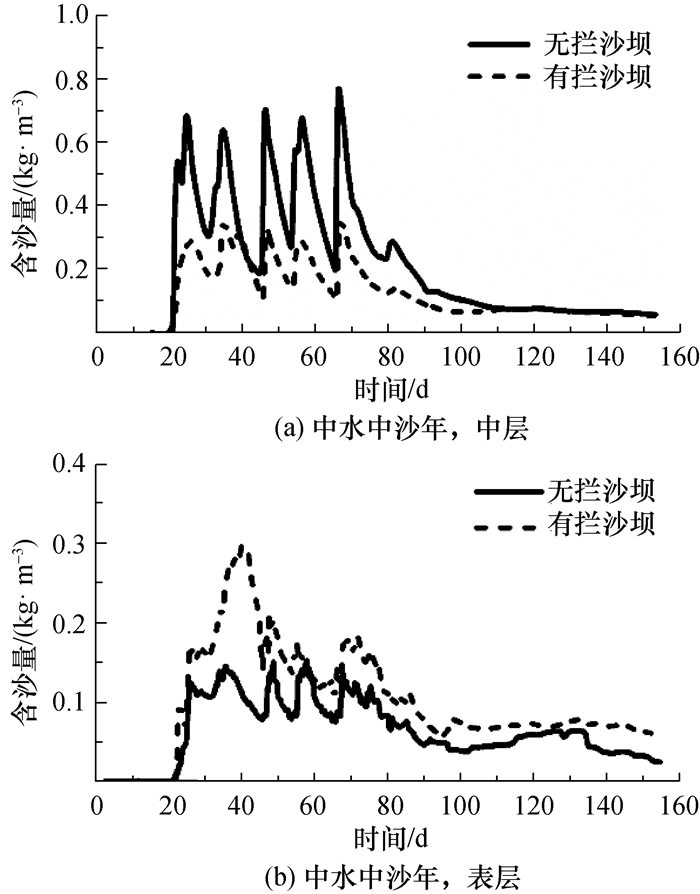图 11 有、无拦沙坝条件下中水中沙年中、表层含沙量分布

 $S=0.001156 \times \mathrm{TU}+0.03652.$ (13)

 % 位置 浊度区间/NTU 大水大沙年 中水中沙年 小水小沙年 无坝 有坝 增加 无坝 有坝 增加 无坝 有坝 增加 中层 < 17.1 16.6 18.5 2.0 13.5 13.9 0.4 10.8 18.5 7.7 17.1~100 21.8 52.7 30.9 41.7 55.0 13.3 56.4 42.6 -13.8 >100 61.6 28.7 -32.9 44.7 31.0 -13.7 32.8 38.9 6.0 表层 < 17.1 47.0 23.0 -24.0 48.0 13.6 -34.4 62.1 14.6 -47.5 17.1~100 45.4 50.2 4.9 52.0 62.9 10.9 30.8 69.1 38.3 >100 7.6 26.7 19.1 0.0 23.5 23.5 7.1 16.3 9.2

3 结论

1) 现状条件下取水口断面的汛期含沙量具有明显的分层特点，表层水体的浊度有47.0%~62.1%的时间达到小于17.1 NTU的要求，其余时间的浊度虽然不能达标，但仅有0%~7.6%的时间大于100 NTU。而中层水体的浊度只有10.8%~16.6%的时间小于17.1 NTU，有32.8%~61.1%的时间大于100 NTU。可见与现有中层取水口相比，新建分层取水口方案充分利用了取水口含沙量的分层特点，在提高汛期取水浊度满足要求的时间上有明显优势，可作为牛栏江滇池补水工程汛期取水防沙措施的首选方案。

2) 修建拦沙坝可促进泥沙在拦沙坝以上库区的淤积，明显降低取水口断面的汛期平均含沙量和中层含沙量，将平均含沙量从0.21~0.28 kg/m3降至0.14~0.19 kg/m3，中层水体浊度大于100 NTU的时间比例从32.8%~61.6%降低至28.7%~38.9%。但降低后的中层含沙量仍偏高，只能将小于17.1 NTU的占比从10.8%~16.6%提升到13.9%~18.5%，效果并不明显。本研究结果说明拦沙坝方案能缓解汛期水体浑浊对滇池水环境及瀑布公园和盘龙江水景观的影响，但对提高取水浊度满足要求时间的作用有限。

3) 由于拦沙坝对泥沙垂向分层的扰动，导致取水口含沙量的垂向分布趋于均匀，表层水体浊度增加，其小于17.1 NTU的时间比例从47.0%~62.1%降低至13.6%~23.0%，拦沙坝与分层取水方式之间存在矛盾，需要注意。

  刘绍川, 杨吉健, 李浩东.牛栏江干河泵站取水防沙治理一期工程可行性研究报告[R].昆明: 中国电建昆明勘测设计研究院有限公司, 2016. LIU S C, YANG J J, LI H D. Feasibility study report on the first-stage project of the water intake and sand control of the pump station in the Niulan River and Gan River[R]. PowerChina Kunming Engineering Corporation Limited, 2016. (in Chinese)  吴保生, 张俊华, 曲少军, 等.牛栏江干河泵站汛期取水防沙治理研究报告[R].北京: 清华大学, 2018. WU B S, ZHANG J H, QU S J, et al. Study report on sediment preventing measures for the water intake and sand control of the pump station in the Niulan River and Gan River in flood seasons[R]. Beijing: Tsinghua University, 2018. (in Chinese)  朱鉴远. 水利水电工程泥沙设计[M]. 北京: 中国水利水电出版社, 2010. ZHU J Y. Sediment design for hydraulic engineering[M]. Beijing: China Water & Power Press, 2010. (in Chinese)  中国水利学会泥沙专业委员会. 泥沙手册[M]. 北京: 中国环境科学出版社, 1992. Sediment commission of China water conservancy association. Sediment manual[M]. Beijing: China Environmental Science Press, 1992. (in Chinese)  张少雄.大型水库分层取水下泄水温研究[D].天津: 天津大学, 2012. ZHANG S X. Study on temperature of water released by selective withdrawal from large reservoirs[D]. Tianjin: Tianjin University, 2012. (in Chinese) http://cdmd.cnki.com.cn/Article/CDMD-10056-1013004930.htm  范志国, 郑向晖, 刘国军, 等. 新型分层取水结构的研究与应用[J]. 水利水电技术, 2017, 48(10): 103-108, 128. FAN Z G, ZHENG X H, LIU G J, et al. Study and application of new type multi-level intake structure[J]. Water Resources and Hydropower Engineering, 2017, 48(10): 103-108, 128. (in Chinese)  ŞEBNEM E. Effects of thermal stratification and mixing on reservoir water quality[J]. Limnology, 2008, 9(2): 135-142. DOI:10.1007/s10201-008-0240-x  MILSTEIN A, ZORAN M. Effect of water withdrawal from the epilimnion on thermal stratification in deep dual purpose reservoirs for fish culture and field irrigation[J]. Aquaculture International, 2001, 9(1): 81-86. DOI:10.1023/A:1012530409312  钱宁, 万兆惠. 泥沙运动力学[M]. 北京: 科学出版社, 1983. QIAN N, WAN Z H. Mechanics of sediment transport[M]. Beijing: Science Press, 1983. (in Chinese)  LIEN H P. Design of slit dams for controlling stony debris flows[J]. International Journal of Sediment Research, 2003, 18(1): 74-87.  贾世涛, 崔鹏, 陈晓清, 等. 拦沙坝调节泥石流拦挡与输移性能的试验研究[J]. 岩石力学与工程学报, 2011, 30(11): 2338-2345. JIA S T, CUI P, CHEN X Q, et al. Experimental study of regulating barrage and transportation properties of debris flow by silt-trap dam[J]. Chinese Journal of Rock Mechanics and Engineering, 2011, 30(11): 2338-2345. (in Chinese)  况文军, 孟天友, 杨秀才. 拦沙坝、谷坊在赫章县水土保持防护体系中的作用[J]. 中国水土保持, 2007(8): 44-45. KUANG W J, MENG T Y, YANG X C. The effects of check dams on the water and soil conservation in Hezhang County[J]. Soil and Water Conservation in China, 2007(8): 44-45. DOI:10.3969/j.issn.1000-0941.2007.08.018 (in Chinese)  曹成, 李凤梅. 拦沙坝对小流域水土保持能力调控研究[J]. 水土保持应用技术, 2015(3): 5-6. CAO C, LI F M. Study on the functions of check dams on regulating the water and soil conservation capacity[J]. Technology of Soil and Water Conservation, 2015(3): 5-6. DOI:10.3969/j.issn.1673-5366.2015.03.02 (in Chinese)  李希霞, 王延贵, 吕秀贞. 板桥峪抽水蓄能电站防沙措施的试验研究[J]. 泥沙研究, 2002(4): 30-34. LI X X, WANG Y G, LU X Z. Experimental study on sediment preventing measures for Banqiaoyu pumped storage hydroplant[J]. Journal of Sediment Research, 2002(4): 30-34. DOI:10.3321/j.issn:0468-155X.2002.04.006 (in Chinese)  ZHANG Y, BAPTISTA A M. SELFE:A semi-implicit Eulerian-Lagrangian finite-element model for cross-scale ocean circulation[J]. Ocean Modelling, 2008, 21: 71-96. DOI:10.1016/j.ocemod.2007.11.005  ZHANG Y J, YE F, STANEV E V, et al. Seamless cross-scale modeling with SCHISM[J]. Ocean Modelling, 2016, 102: 64-81. DOI:10.1016/j.ocemod.2016.05.002  YE F, ZHANG Y J, FRIEDRICHS M A M, et al. A 3-D, cross-scale, baroclinic model with implicit vertical transport for the Upper Chesapeake Bay and its tributaries[J]. Ocean Modelling, 2016, 107: 82-96. DOI:10.1016/j.ocemod.2016.10.004  ZHANG Y J, STANEV E V, GRASHORN S. Unstructured-grid model for the North Sea and Baltic Sea:Validation against observations[J]. Ocean Modelling, 2016, 97: 91-108. DOI:10.1016/j.ocemod.2015.11.009  AZEVEDO A, OLIVEIRA A, FORTUNATO A B, et al. A cross-scale numerical modeling system for management support of oil spill accidents[J]. Marine Pollution Bulletin, 2014, 80: 132-147. DOI:10.1016/j.marpolbul.2014.01.028  李肖男.黄河三维水沙数学模型研究及应用[D].北京: 清华大学, 2017. LI X N. Study and application of the three-dimensional numerical model for water-sediment transport in the Yellow River[D]. Beijing: Tsinghua University, 2017. (in Chinese)  PINTO L, FORTUNATO A B, ZHANG Y, et al. Development and validation of a three-dimensional morphodynamic modelling system for non-cohesive sediments[J]. Ocean Modelling, 2012, 57-58(11): 1-14.  WU W, RODI W, WENKA T. 3-D numerical modeling of flow and sediment transport in open channels[J]. Journal of Hydraulic Engineering, 2000, 126(1): 4-15. DOI:10.1061/(ASCE)0733-9429(2000)126:1(4)  ZHANG H, HUANG Y, ZHAO L. A mathematical model for unsteady sediment transport in the Lower Yellow River[J]. International Journal of Sediment Research, 2001, 16(2): 150-158.  张红武, 张清. 黄河水流挟沙力的计算公式[J]. 人民黄河, 1992(11): 7-9. ZHANG H W, ZHANG Q. Formula of sediment carrying capacity of the Yellow River[J]. Yellow River, 1992(11): 7-9. (in Chinese)  李义天. 冲淤平衡状态下床沙质级配初探[J]. 泥沙研究, 1987(1): 82-87. LI Y T. Preliminary study on the bed material gradation under the balanced state of silting and scoring[J]. Journal of Sediment Research, 1987(1): 82-87. (in Chinese)  SONG Y, HAIDVOGEL D. A semi-implicit ocean circulation model using a generalized topography-following coordinate system[J]. Journal of Computational Physics, 1994, 115(1): 228-244.  张瑞瑾. 河流泥沙动力学[M]. 2版. 北京: 中国水利水电出版社, 1998. ZHANG R J. Mechanics of sediment transport[M]. 2nd ed. Beijing: China Water & Power Press, 1998. (in Chinese)